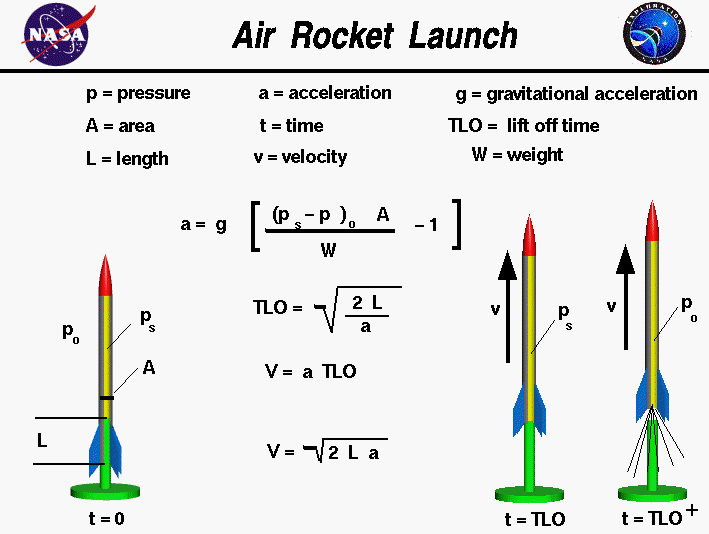+ Text Only Site
+ Non-Flash Version
+ Contact GlennFlying model rockets is a relatively safe and inexpensive way for students to learn the basics of forces and the response of a vehicle to external forces. A model rocket is subjected to four forces in flight; weight, thrust, and the aerodynamic forces, lift and drag. There are many different types of model rockets. The simplest type of rocket that a student encounters is the compressed air rocket. The air rocket system consists of two main parts, the launcher and the rocket. On this page we show the equations which model the launch of a compressed air rocket. On the figure we show a generic launcher, although launchers come in a wide variety of shapes and sizes. The launcher has a base to support the rocket during launch and a hollow launch tube mounted perpendicular to the base. The launch tube is inserted into the base of the rocket before launch and forms a closed pressure vessel with the sides and nose cone of the rocket. We have cut the rocket along its axis so that you can see the launch tube inside the body of the rocket; the inside of the rocket is colored yellow to the right, the outside surface of the rocket is in silver to the left,and the launch tube is colored green at the bottom. We will denote the length of the launch tube inside the body of the rocket by the symbol L. The launch tube is connected to an air pump which is used to increase the pressure inside the body tube. We will denote the launch pressure by the symbol ps. The pressure exerts an equal force on all sides of the pressure vessel. Because the rocket is free to move along the launch tube, the pressure exerts a vertical force given by: Fp = (ps - po) * A where Fp is the pressure force, po is the atmospheric pressure, and A is the cross-sectional area of the rocket tube. The net force acting on the rocket is the difference of the vertical pressure force and the weight W of the rocket: Fnet = (ps - po) * A - W To simplify the analysis, we are going to assume that the length of the launch tube is small relative to the length of the rocket. As the rocket moves, the pressure remains fairly constant inside the tube. In reality, there is a slight decrease in pressure as the volume increase, but we are going to neglect that effect to simplify this analysis. With a constant pressure, and constant pressure force, we can use a simple algebraic form of Newton's second law to determine the acceleration a, velocity v and the distance y which the rocket moves along the launch tube: Fnet = m * a m * a = (ps - po) * A - W where m is the mass of the rocket This equation can also be written as: W * a = g * ((ps - po) * A - W) where g is the gravitational acceleration, which is equal to 32.2 ft/sec^2 or 9.8 m/sec^2 on the surface of the Earth. The value of g is different on the Moon, and on Mars. The weight W is equal to the mass times the gravitational acceleration. Now divide both sides by the weight to determine the rocket acceleration along the launch tube: a = g * ((ps - po) * A / W - 1) For a constant acceleration, the velocity v and distance traveled y are given by: v = a * t y = .5 * a * t^2 where t is the time. Using the length of the launch tube L, we can now solve for the lift off time - TLO by using the second equation: TLO = sqrt (2 * L / a) and then using this time, we can use the first equation to solve for the velocity at the end of the tube: v = TLO * a v = sqrt ( 2 * L * a) As the rocket clears the end of the launch tube (t = TLO+), the air inside the rocket, at pressure ps, will rush out the exit and the pressure will equalize with free stream inside the tube. In reality, this will provide an additional amount of thrust T given by: T = m dot * Ve where m dot is the air mass flow rate out of the tube, Ve is the exit velocity of the air. For an air rocket, with no nozzle and small volume, this additional thrust is small relative to the weight of the rocket, and we will ignore this effect. The velocity v at the end of the launch tube is used as the initial velocity in the flight equations to determine the flight trajectory of a compressed air rocket. You can study the flight of a stomp rocket by using the RocketModeler III simulation program. And here is an interactive, JavaScript simulation of the launch process: Stomp You can launch the air rocket by using the buttons at the bottom of the simulator. "Reset" brings the rocket back to its original configuration. Guided ToursCompressed Air Rocket:RocketModeler III:Activities: Related Sites: Rocket Index Rocket Home Beginner's Guide Home+ Inspector General Hotline + Equal Employment Opportunity Data Posted Pursuant to the No Fear Act + Budgets, Strategic Plans and Accountability Reports + Freedom of Information Act + The President's Management Agenda + NASA Privacy Statement, Disclaimer, and Accessibility CertificationEditor: Daniel Rodriguez Lopez NASA Official: Nancy Hall Last Updated: May 13 2021 + Contact Glenn